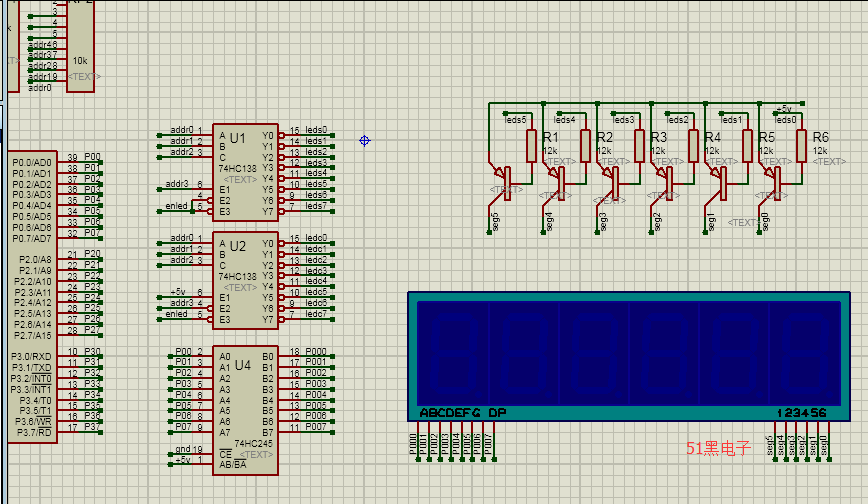##帐号 自动登录 找回密码 密码 立即注册

# 单片机数码管显示数字程序 请赐教ID:1054848 发表于 2022-12-1 14:34 | 显示全部楼层 |阅读模式
 我的C代码和仿真放在RAR中了。请赐教单片机源程序如下: #include sbit ADDR2 =P1^2; sbit ADDR1 =P1^1; sbit ADDR0 =P1^0; sbit ENLED =P1^4; sbit ADDR3 =P1^3; unsigned char code LedChar=        //数码管状态值初始化 {0xC0, 0xF9, 0xA4, 0xB0, 0x99, 0x92, 0x82, 0xF8, 0x80, 0x90, 0x88, 0x83, 0xC6, 0xA1, 0x86, 0x8E}; void main() {       unsigned int i=0, x;     unsigned char Second=0;//定义一个一秒之后自增1的变量     ADDR3=1;//使能三八译码器     ENLED=0;//         while(1)     {            for(x=0; x<20627; x++)//软件调试得出这个循环耗时1秒         {             P0=0xFF;//P0端口状态全部熄灭数码管里的LED达到刷新作用             switch(i)             {                 case 0:                 ADDR2=0; ADDR1=0; ADDR0=0; P0=LedChar[Second%10];       i++; break;                 case 1:                 ADDR2=0; ADDR1=0; ADDR0=1; P0=LedChar[(Second/10)%10];  i++; break;                      case 2:                 ADDR2=0; ADDR1=1; ADDR0=0; P0=LedChar[(Second/100)%10]; i=0; break;               }           }         Second++;//一秒之后变量自加1     } } //=========================================================== //C语言网 /*栏目----单片机编程----第四章 数码管*/ //同时显示不同的数字 /* 在上一讲的代码中几个数码管只能同时显示相同的数字和字母，这一讲我们实现同时3个数码管点亮但每个数码管显示的数字都不一样，所以我们做一个用来显示一个变量一秒后自加1的显示效果。 例如，此时时间过去了162秒 数码管2 1.取余 先定义一个8位的变量Second，最多能计到255秒，所以我们只用到3个数码管而已，这3个数码管的显示只能显示0~9。 这里我们首先来了解一下取余。 162%10=2; (162/10)%10=6; (162/100)%10=1; 大家要知道Second是unsigned char无符号整型变量,比如当Second等于169时，(Second/10)是等于16的，后面的小数直接舍去，就算小数是9也照样舍去，没有四舍五入的说法。 到这里我们也就不难理解，当Second为162时，分别让3个数码管显示各自的位数，数码管2显示百位数1，数码管1显示十位数6，数码管0显示个位数2。 2.代码分析 首先要同时点亮3个数码管就必须让三八译码器的IO0~IO2轮流快速输出低电平，然后在数码管0被点亮的瞬间之后P0被赋值为LedChar[Second%10]，这样数码管0就显示了这个变量的个位数。 然后又到了数码管1被点亮，马上P0被赋值为LedChar[(Second/10)%10]，数码管1显示了这个变量的十位数。 接着就是到了数码管2被点亮，马上P0被赋值为LedChar[(Second/100)%10]，数码管2显示了这个变量的百位数。 大家要有一个意识，那就是随着每次IO0, IO1, IO2输出低电平之后的瞬间，P0都会改变它8个IO端口输出状态以显示不同的数字。 3.代码 #include sbit ADDR2=P1^2; sbit ADDR1=P1^1; sbit ADDR0=P1^0; sbit ENLED=P1^4; sbit ADDR3=P1^3; unsigned char code LedChar={0xC0, 0xF9, 0xA4, 0xB0, 0x99, 0x92, 0x82, 0xF8, 0x80, 0x90, 0x88, 0x83, 0xC6, 0xA1, 0x86, 0x8E};//数码管状态值初始化 void main() {       unsigned int i=0, x;     unsigned char Second=0;//定义一个一秒之后自增1的变量     ADDR3=1;//使能三八译码器     ENLED=0;//         while(1)     {            for(x=0; x<20627; x++)//软件调试得出这个循环耗时1秒         {             P0=0xFF;//P0端口状态全部熄灭数码管里的LED达到刷新作用             switch(i)             {                 case 0:                 ADDR2=0; ADDR1=0; ADDR0=0; P0=LedChar[Second%10];       i++;break;                        case 1:                 ADDR2=0; ADDR1=0; ADDR0=1; P0=LedChar[(Second/10)%10];  i++;break;                             case 2:                 ADDR2=0; ADDR1=1; ADDR0=0; P0=LedChar[(Second/100)%10]; i=0; break;               }           }         Second++;//一秒之后变量自加1     } } 4.代码实现原理 我们知道for循环的持续时间为1秒，我们拆开代码分析就像这样 第一次for循环执行的内容为 P0=0xFF; ADDR2=0; ADDR1=0; ADDR0=0; P0=LedChar[Second%10];i++; 第二次for循环执行的内容为 P0=0xFF; ADDR2=0; ADDR1=0; ADDR0=1; P0=LedChar[(Second/10)%10];i++; 第三次for循环执行的内容为 P0=0xFF; ADDR2=0; ADDR1=1; ADDR0=0; P0=LedChar[(Second/100)%10];i=0; 一直循环下去，也就达到了三八译码器IO0~IO2轮流快速地切换输出低电平了，也就做到同时点亮的作用，直到for循环持续的时间结束就“Second++;”但这条语句执行地很快于是又重新for循环了，但是Second却改变了数字，LedChar[Second%10]也就不是之前的状态值了。 */复制代码27.92 KB, 下载次数: 6, 下载积分: 黑币 -5ID:262 发表于 2022-12-1 16:56 | 显示全部楼层
 程序的注释很详细 感谢您的赐教

 本版积分规则 回帖后跳转到最后一页